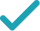Type a math problemSolve for KSteps for Solving Linear Equation
Multiply both sides of the equation by .
Multiply and to get .
Multiply and to get .
Swap sides so that all variable terms are on the left hand side.
The equation is in standard form.
Divide both sides by .
Dividing by undoes the multiplication by .
Divide by .
Solve for T_2Solution Steps
Multiply and to get .
Multiply and to get .
Cancel out in both numerator and denominator.
Multiply and to get .
Divide by to get .
Solve for mSteps by Finding Square Root
Steps by Finding Square Root
Variable cannot be equal to since division by zero is not defined. Multiply both sides of the equation by .
Multiply and to get .
Multiply and to get .
Subtract from both sides.
Combine all terms containing .
Divide both sides by .
Dividing by undoes the multiplication by .
Divide by .
Take the square root of both sides of the equation.
The equation is now solved. Solutions are the same.
Variable cannot be equal to .
Assign T_2Giving is as easy as 1, 2, 3
Get 1,000 points to donate to a school of your choice when you join Give With Bing
T_{2}\times 380m^{2}=1.52mm\times 290K
Multiply both sides of the equation by 380m^{2}.
T_{2}\times 380m^{2}=1.52m^{2}\times 290K
Multiply m and m to get m^{2}.
T_{2}\times 380m^{2}=440.8m^{2}K
Multiply 1.52 and 290 to get 440.8.
440.8m^{2}K=T_{2}\times 380m^{2}
Swap sides so that all variable terms are on the left hand side.
\frac{2204m^{2}}{5}K=380T_{2}m^{2}
The equation is in standard form.
\frac{5\times \left(\frac{2204m^{2}}{5}\right)K}{2204m^{2}}=\frac{5\times 380T_{2}m^{2}}{2204m^{2}}
Divide both sides by 440.8m^{2}.
K=\frac{5\times 380T_{2}m^{2}}{2204m^{2}}
Dividing by 440.8m^{2} undoes the multiplication by 440.8m^{2}.
K=\frac{25T_{2}}{29}
Divide 380T_{2}m^{2} by 440.8m^{2}.
T_{2}=\frac{1.52m^{2}\times 290K}{380mm}
Multiply m and m to get m^{2}.
T_{2}=\frac{1.52m^{2}\times 290K}{380m^{2}}
Multiply m and m to get m^{2}.
T_{2}=\frac{1.52\times 29K}{38}
Cancel out 10m^{2} in both numerator and denominator.
T_{2}=\frac{44.08K}{38}
Multiply 1.52 and 29 to get 44.08.
T_{2}=1.16K
Divide 44.08K by 38 to get 1.16K.
T_{2}\times 380m^{2}=1.52mm\times 290K
Variable m cannot be equal to 0 since division by zero is not defined. Multiply both sides of the equation by 380m^{2}.
T_{2}\times 380m^{2}=1.52m^{2}\times 290K
Multiply m and m to get m^{2}.
T_{2}\times 380m^{2}=440.8m^{2}K
Multiply 1.52 and 290 to get 440.8.
T_{2}\times 380m^{2}-440.8m^{2}K=0
Subtract 440.8m^{2}K from both sides.
\left(T_{2}\times 380-440.8K\right)m^{2}=0
Combine all terms containing m.
\frac{\left(-\frac{2204K}{5}+380T_{2}\right)m^{2}}{-\frac{2204K}{5}+380T_{2}}=\frac{0}{-\frac{2204K}{5}+380T_{2}}
Divide both sides by 380T_{2}-440.8K.
m^{2}=\frac{0}{-\frac{2204K}{5}+380T_{2}}
Dividing by 380T_{2}-440.8K undoes the multiplication by 380T_{2}-440.8K.
m^{2}=0
Divide 0 by 380T_{2}-440.8K.
m=0 m=0
Take the square root of both sides of the equation.
m=0
The equation is now solved. Solutions are the same.
m\in \emptyset
Variable m cannot be equal to 0.
T_{2}\times 380m^{2}=1.52mm\times 290K
Variable m cannot be equal to 0 since division by zero is not defined. Multiply both sides of the equation by 380m^{2}.
T_{2}\times 380m^{2}=1.52m^{2}\times 290K
Multiply m and m to get m^{2}.
T_{2}\times 380m^{2}=440.8m^{2}K
Multiply 1.52 and 290 to get 440.8.
T_{2}\times 380m^{2}-440.8m^{2}K=0
Subtract 440.8m^{2}K from both sides.
\left(T_{2}\times 380-440.8K\right)m^{2}=0
Combine all terms containing m.
\left(-\frac{2204K}{5}+380T_{2}\right)m^{2}=0
Quadratic equations like this one, with an x^{2} term but no x term, can still be solved using the quadratic formula, \frac{-b±\sqrt{b^{2}-4ac}}{2a}, once they are put in standard form: ax^{2}+bx+c=0.
m=\frac{0±\sqrt{0^{2}}}{2\left(-\frac{2204K}{5}+380T_{2}\right)}
This equation is in standard form: ax^{2}+bx+c=0. Substitute 380T_{2}-440.8K for a, 0 for b, and 0 for c in the quadratic formula, \frac{-b±\sqrt{b^{2}-4ac}}{2a}.
m=\frac{0±0}{2\left(-\frac{2204K}{5}+380T_{2}\right)}
Take the square root of 0^{2}=0.
m=\frac{0}{-\frac{4408K}{5}+760T_{2}}
Multiply 2 times 380T_{2}-440.8K.
m=0
Divide 0 by 760T_{2}-\frac{4408K}{5}.
m\in \emptyset
Variable m cannot be equal to 0.